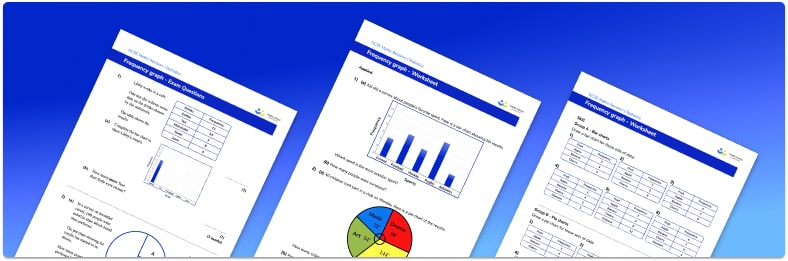# Frequency Graph Worksheet• Section 1 of the frequency graph worksheet contains 30 skills-based frequency graph questions, in 3 groups to support differentiation
• Section 2 contains 3 applied frequency graph questions with a mix of worded problems and deeper problem solving questions
• Section 3 contains 3 foundation and higher level GCSE exam style frequency graph questions
• Answers and a mark scheme for all frequency graph questions are provided
• Questions follow variation theory with plenty of opportunities for students to work independently at their own level
• All questions created by fully qualified expert secondary maths teachers
• Suitable for GCSE maths revision for AQA, OCR and Edexcel exam boards

• This field is for validation purposes and should be left unchanged.

You can unsubscribe at any time (each email we send will contain an easy way to unsubscribe). To find out more about how we use your data, see our privacy policy.

### Frequency graph at a glance

A frequency is the number of times something occurs (the number of hours on social media, the number of each different flavoured sweet in a packet, the number of cars in a car park, etc). A frequency graph represents the frequencies for a data set in a visual way. There are many types of frequency graphs including varieties of bar charts (standard, comparative, and compound), line graphs (including time series), vertical line graphs, and pie charts.

For most frequency graphs for discrete data, the horizontal axis is labelled with the categories, and the vertical axis is always labelled as frequency; pie charts are not drawn on a set of axes and so the size of the sector is proportional to the frequency of that category.

Frequency graphs for grouped data or continuous data include cumulative frequency diagrams and histograms. With these two types of frequency graphs, the frequency for the class interval is calculated using the graph as the frequency is not immediately visible.

The horizontal axis for cumulative frequency graphs and histograms is a continuous scale. The vertical axis for a cumulative frequency graph is labelled as the cumulative frequency (the running total) and the vertical axis for a histogram is labelled as the frequency density.

We can calculate averages such as the median, state or estimate maximum values and minimum values for a set of data, state the upper quartile, lower quartile and by subtracting them, calculate the interquartile range, depending on the type of data we are analysing. We can also look at the frequency distribution for a set of data, looking at the spread, skewness and outliers using frequency graphs.

Looking forward, students can then progress to additional Statistics worksheets, for example a mean, median, mode and range worksheet, the cumulative frequency curve worksheet, or the

For more teaching and learning support on Statistics our GCSE maths lessons provide step by step support for all GCSE maths concepts.

## Do you have KS4 students who need more focused attention to succeed at GCSE?There will be students in your class who require individual attention to help them succeed in their maths GCSEs. In a class of 30, it’s not always easy to provide.

Help your students feel confident with exam-style questions and the strategies they’ll need to answer them correctly with our dedicated GCSE maths revision programme.

Lessons are selected to provide support where each student needs it most, and specially-trained GCSE maths tutors adapt the pitch and pace of each lesson. This ensures a personalised revision programme that raises grades and boosts confidence.

Find out more